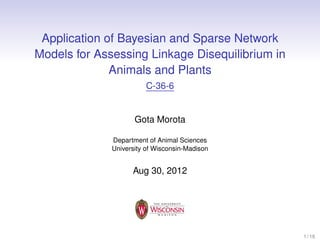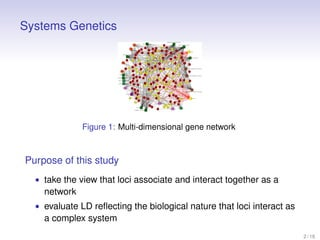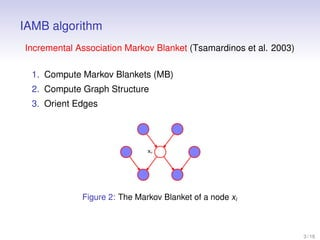Successfully reported this slideshow.

# Application of Bayesian and Sparse Network Models for Assessing Linkage Disequilibrium in Animals and Plants

0

Share×
1 of 15
1 of 15

# Application of Bayesian and Sparse Network Models for Assessing Linkage Disequilibrium in Animals and Plants

0

Share

Presented at 26th International Biometric Conference. August 26-31, Kobe, Japan.

Presented at 26th International Biometric Conference. August 26-31, Kobe, Japan.

## More Related Content

### Related Books

Free with a 14 day trial from Scribd

See all

### Related Audiobooks

Free with a 14 day trial from Scribd

See all

### Application of Bayesian and Sparse Network Models for Assessing Linkage Disequilibrium in Animals and Plants

1. 1. Application of Bayesian and Sparse Network Models for Assessing Linkage Disequilibrium in Animals and Plants C-36-6 Gota Morota Department of Animal Sciences University of Wisconsin-Madison Aug 30, 2012 1 / 16
2. 2. Systems Genetics Figure 1: Multi-dimensional gene network Purpose of this study • take the view that loci associate and interact together as a network • evaluate LD reﬂecting the biological nature that loci interact as a complex system 2 / 16
3. 3. IAMB algorithm Incremental Association Markov Blanket (Tsamardinos et al. 2003) 1. Compute Markov Blankets (MB) 2. Compute Graph Structure 3. Orient Edges Figure 2: The Markov Blanket of a node xi 3 / 16
4. 4. Identifying the MB of a node • Growing phase • heuristic function: f (X ; T |CMB ) = MI(X ; T |CMB ) = cmb ∈CMB     P (CMB )     P (X , T |CMB )    P (X , T |CMB ) log  P (X |CMB )P (T |CMB )   x ∈X t ∈T • conditional independence tests (Pearson’s χ2 test): H0 : P (X , T |CMB ) = P (X |CMB ) · P (T |CMB ) (do not add X ) HA : P (X , T |CMB ) P (X |CMB ) · P (T |CMB ) (add X to the CMB) • Shrinking phase • conditional independence tests (Pearson’s χ2 test): H0 : P (X , T |CMB − X ) P (X |CMB − X ) · P (T |CMB − X ) (keep X ) HA : P (X , T |CMB − X ) = P (X |CMB − X ) · P (T |CMB − X ) (remove X 4 / 16
5. 5. Network Structure Algorithm Suppose Y ∈ MB (T ). Then T and Y are connected if they are conditionally dependent given all subsets of the smaller of MB (T ) − (Y ) and MB (Y ) − (T ). Example: • MB (T ) = (A , B , Y ), MB (Y ) = (C , D , E , F , T ) • since MB (T ) < MB (Y ), independence tests are conditional on all subsets of MB (T ) − (Y ) = (A , B ). • if any of the CI(T , Y |{}), CI(T , Y |{A }), CI(T , Y |{B }), andCI(T , Y |{A , B }) imply conditional independence, ↓ • T and Y are considered separate (spouses) • repeat for T ∈ S and Y ∈ MB (T ), 5 / 16
6. 6. Materials 1. Data • 4,898 Holstein bulls (USDA-ARS AIPL) • 37,217 SNP markers (MAF > 0.025) • milk protein yield 2. Missing genotypes imputation • fastPHASE (Scheet and Stephens, 2006) 3. Select 15 SNPs • Bayesian LASSO 4. uncover associations among a set of marker loci found to have the strongest effects on milk protein yield 6 / 16
7. 7. Results – Top 15 SNPs IAMB algorithm Pairwise LD among SNPs (r2) J d A c b a Z L Y X M N W V U F T S B K G R Q P H O N O E M L K J I I H G F E C D C B R2 Color Key A 0 Figure 3: r 2 0.2 0.4 0.6 0.8 1 D Figure 4: IAMB 7 / 16
8. 8. Conclusion and Possible Improvements • LD relationships are of a multivariate nature • r 2 gives an incomplete description of LD ⇓ • undirected networks • sparsity 8 / 16
9. 9. Pairwise Binary Markov Networks We estimate the Markov network parameters Θp ×p by maximizing a log-likelihood.      f (x1 , ..., xp ) = exp     Ψ(Θ) 1 p θj ,j xj + j =1 1≤j <k ≤p      θj ,k xj xk     (1) where xj ∈ {0, 1} Ψ(Θ) = x ∈0 , 1 (2)      exp     p θj ,j xj + j =1 1 ≤j <k ≤p      θj ,k xj xk     (3) • the ﬁrst term is a main effect of binary marker xj (node potential) • the second term corresponds to an“interaction effect” between binary markers xj and xk (link potential) • Ψ(Θ) is the normalization constant (partition function) 9 / 16
10. 10. Ravikumar et al. (2010) The pseudo-likelihood based on the local conditional likelihood associated with each binary marker can be represented as n p x φi ,ij,j (1 − φi ,j )1−xi,j l (Θ) = (4) i =1 j =1 where φi ,j is the conditional probability of xi ,j = 1 given all other variables. Using a logistic link function, φi ,j = P(xi ,j = 1|xi ,k , k j ; θj ,k , 1 ≤ k ≤ p ) exp(θj ,j + k j θj ,k xi ,k ) = 1 + exp(θj ,j + k j θj ,k xi ,k ) (5) (6) 10 / 16
11. 11. Ravikumar et al. (2010) (cont.) • L1 regularized logistic regressions problem • regressing each marker on the rest of the markers • the network structure is recovered from the sparsity pattern of the regression coefﬁcients   0    ˆ−2  β   1     ˆ  .  . Θ= .    −(p −1)  ˆ β   1   −p  ˆ β1 ˆ β −1 , 2 0 ··· , ··· , ··· , 0 ˆ−(p −1) · · · , β p −2 ˆ p · · · , β−−2 p ˜ Θ= ˆ ˆ Θ • ΘT ˆ 1 β−−1 p ˆ 2 β−−1 p ˆp β −1 ˆp β −2 . . .                ··· ,     −(p −1)   ˆp  0 β     −p ˆ β p −1 0 (7) (8) 11 / 16
12. 12. Materials 1. Data • 599 inbred wheat lines (CIMMYT) • 1447 Diversity Array Technology (DArT) binary markers • mean grain yields 2. Select 30 SNPs • Bayesian LASSO 3. Benchmark methods • IAMB algorithm • r2 12 / 16
13. 13. lambda = CV 9 8 7 Bayesian Network 9 6 10 8 7 6 10 5 11 4 3 12 5 11 13 4 3 12 13 2 2 14 1 14 15 0 15 0 16 29 16 29 28 17 18 27 19 26 20 25 21 22 23 24 Figure 5: L1 regularization 1 28 17 18 27 19 26 20 25 21 22 23 24 Figure 6: IAMB 13 / 16
14. 14. Summary Interactions and associations among the cells and genes form a complex biological system ⇓ • r 2 → association(m1, m2)|∅ (empty set) • L1 regularized MN → association(m1, m2) | else A ﬁnal remark • selecting tag SNPs unconditionally, as well as conditionally, on other markers when the dimension of the data is high • data generated from next generation sequence technologies 14 / 16
15. 15. Acknowledgments University of Wisconsin-Madison • Daniel Gianola • Guilherme Rosa University College London • Marco Scutari • Kent Weigel • Bruno Valente 15 / 16